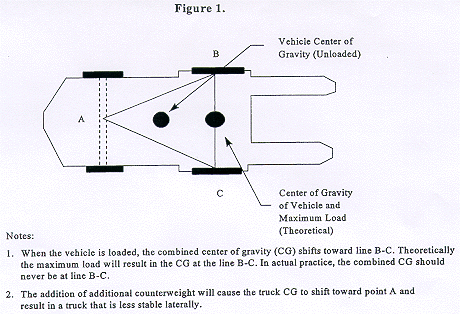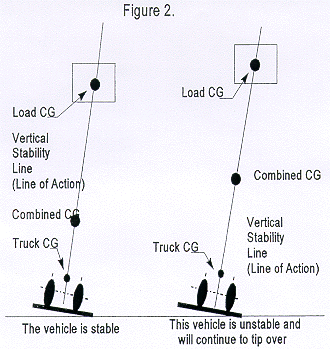• Part Number:
1910
• Part Number Title:
Occupational Safety and Health Standards
• Subpart:
1910 Subpart N
• Subpart Title:
Materials Handling and Storage
• Standard Number:
• Title:
Stability of Powered Industrial Trucks (Non-mandatory Appendix to Paragraph (l) of This Section)
• GPO Source:

Appendix A - Stability of Powered Industrial Trucks (Non-mandatory Appendix to Paragraph (l) of This Section)

A-1. Definitions. The following definitions help to explain the principle of stability:

Center of gravity is the point on an object at which all of the object's weight is concentrated. For symmetrical loads, the center of gravity is at the middle of the load.

Counterweight is the weight that is built into the truck's basic structure and is used to offset the load's weight and to maximize the vehicle's resistance to tipping over.

Fulcrum is the truck's axis of rotation when it tips over.

Grade is the slope of a surface, which is usually measured as the number of feet of rise or fall over a hundred foot horizontal distance (the slope is expressed as a percent).

Lateral stability is a truck's resistance to overturning sideways.

Line of action is an imaginary vertical line through an object's center of gravity.

Load center is the horizontal distance from the load's edge (or the fork's or other attachment's vertical face) to the line of action through the load's center of gravity.

Longitudinal stability is the truck's resistance to overturning forward or rearward.

Moment is the product of the object's weight times the distance from a fixed point (usually the fulcrum). In the case of a powered industrial truck, the distance is measured from the point at which the truck will tip over to the object's line of action. The distance is always measured perpendicular to the line of action.

Track is the distance between the wheels on the same axle of the truck.

Wheelbase is the distance between the centerline of the vehicle's front and rear wheels.

A-2. General.

A-2.1. Determining the stability of a powered industrial truck is simple once a few basic principles are understood. There are many factors that contribute to a vehicle's stability: the vehicle's wheelbase, track, and height; the load's weight distribution; and the vehicle's counterweight location (if the vehicle is so equipped).

A-2.2. The "stability triangle," used in most stability discussions, demonstrates stability simply.

A-3. Basic Principles.

A-3.1. Whether an object is stable depends on the object's moment at one end of a system being greater than, equal to, or smaller than the object's moment at the system's other end. This principle can be seen in the way a see-saw or teeter-totter works: that is, if the product of the load and distance from the fulcrum (moment) is equal to the moment at the device's other end, the device is balanced and it will not move. However, if there is a greater moment at one end of the device, the device will try to move downward at the end with the greater moment.

A-3.2. The longitudinal stability of a counterbalanced powered industrial truck depends on the vehicle's moment and the load's moment. In other words, if the mathematic product of the load moment (the distance from the front wheels, the approximate point at which the vehicle would tip forward) to the load's center of gravity times the load's weight is less than the vehicle's moment, the system is balanced and will not tip forward. However, if the load's moment is greater than the vehicle's moment, the greater load-moment will force the truck to tip forward.

A-4. The Stability Triangle.

A-4.1. Almost all counterbalanced powered industrial trucks have a three-point suspension system, that is, the vehicle is supported at three points. This is true even if the vehicle has four wheels. The truck's steer axle is attached to the truck by a pivot pin in the axle's center. When the points are connected with imaginary lines, this three-point support forms a triangle called the stability triangle. Figure 1 depicts the stability triangle.A-4.2. When the vehicle's line of action, or load center, falls within the stability triangle, the vehicle is stable and will not tip over. However, when the vehicle's line of action or the vehicle/load combination falls outside the stability triangle, the vehicle is unstable and may tip over. (See Figure 2.)A-5. Longitudinal Stability.

A-5.1. The axis of rotation when a truck tips forward is the front wheels' points of contact with the pavement. When a powered industrial truck tips forward, the truck will rotate about this line. When a truck is stable, the vehicle-moment must exceed the load-moment. As long as the vehicle-moment is equal to or exceeds the load-moment, the vehicle will not tip over. On the other hand, if the load moment slightly exceeds the vehicle-moment, the truck will begin to tip forward, thereby causing the rear to lose contact with the floor or ground and resulting in loss of steering control. If the load-moment greatly exceeds the vehicle moment, the truck will tip forward.

A-6. Lateral Stability.

A-6.1. The vehicle's lateral stability is determined by the line of action's position (a vertical line that passes through the combined vehicle's and load's center of gravity) relative to the stability triangle. When the vehicle is not loaded, the truck's center of gravity location is the only factor to be considered in determining the truck's stability. As long as the line of action of the combined vehicle's and load's center of gravity falls within the stability triangle, the truck is stable and will not tip over. However, if the line of action falls outside the stability triangle, the truck is not stable and may tip over. Refer to Figure 2.

A-6.2. Factors that affect the vehicle's lateral stability include the load's placement on the truck, the height of the load above the surface on which the vehicle is operating, and the vehicle's degree of lean.

A-7. Dynamic Stability.

A-7.1. Up to this point, the stability of a powered industrial truck has been discussed without considering the dynamic forces that result when the vehicle and load are put into motion. The weight's transfer and the resultant shift in the center of gravity due to the dynamic forces created when the machine is moving, braking, cornering, lifting, tilting, and lowering loads, etc., are important stability considerations.

A-7.2. When determining whether a load can be safely handled, the operator should exercise extra caution when handling loads that cause the vehicle to approach its maximum design characteristics. For example, if an operator must handle a maximum load, the load should be carried at the lowest position possible, the truck should be accelerated slowly and evenly, and the forks should be tilted forward cautiously. However, no precise rules can be formulated to cover all of these eventualities.

[63 FR 66270, Dec. 1, 1998]